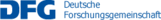## C10 – Sparse controls in optimization of quasilinear partial differential equations

Many practical optimization problems can be described by control problems consisting of  a (often highly nonlinear) partial differential equation (PDE), a so-called objective functional, and possibly further constraints. Questions arising in the context of optimal control problems include the discussion of optimality conditions, convergence of solution algorithms, as well as efficient discretization strategies in e.g. discretization error estimates.  Oftentimes, structural properties allow for a taylored analysis. From the control of simpler linear and semilinear PDEs it is for instance known that for certain objective functionals rather small and simple or sparse structures often emerge as support of an optimal solution. Such sparse structures are also well-suited for practical applications. Mathematical challenges are comparably well understood and analyzed for simpler, linear or semilinear equations, yet less results are available for control of quasilinear, especially parabolic problems. We will analyze and investigate challenges in the presence of quasilinear PDEs.

Name Institute Location Phone
Neitzel, Ira INS FHA7/3.034 69837 neitzel@ins.uni-bonn.de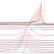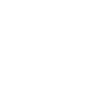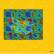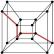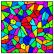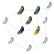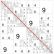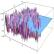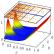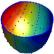## Contact

Coordinator: Prof. Dr. Stefan Müller